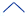﻿ Group functions

Group functions

In SQL, a group function is a function that takes a set (or: group) of multiple column values from multiple rows as input, performs an aggregation, and then returns a single value.

This contrasts with inline functions or scalar functions. They operate on single values by modifying the input value and then returning the modified value.

In the following query, perhaps 100 rows satisfy the WHERE clause. This causes a group of 100 amount values to be passed to MAX(). MAX( ) returns a single value.

 SELECT    MAX( amount ) FROM      order WHERE     month = 'Feb 2020'

The result of this query could be:

 499303900

SQL has 7 group functions:

 Group function Returns Empty-set result value * AVG(  ) The average of the row values passed. The NULL value COUNT(  ) The number of rows passed (independently of values in those rows). 0 (zero) MAX(  ) The greatest of the values passed. The NULL value MIN(  ) The least of the values passed. The NULL value STDDEV(  ) The standard deviation for the set of values passed. The NULL value SUM(  ) The sum of the values passed. The NULL value VARIANCE(  ) The statistical variance for the set of values passed. The NULL value

* The empty-set result value is the value that is the result of applying the group function to an empty row set.Group functions and GROUP BY, HAVINGNULL input valuesNULL output values